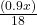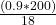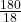## A college received 90% of its order of laptop keyboards from a vendor. Some of the keyboards were defective. The equation below

Question

A college received 90% of its order of laptop keyboards from a vendor.

Some of the keyboards were defective. The equation below represents this

situation, where x represents the total number of keyboards ordered and y

represents the number of keyboards that were non-defective. y = 0.9x –

1/18(0.9x) If the college ordered 200 keyboards, how many non-defective

in progress 0
3 months 2021-07-21T10:03:46+00:00 1 Answers 0 views 0

170

Step-by-step explanation:

Given that: x represents total number of keyboards ordered

y represents number of non-defective keyboards

And;

y = 0.9x –…………………….. 1

If the college ordered 200 keyboards, then x = 200. So that substituting the value of x in equation 1, we have;

y = (0.9 x 200) –= 180 –= 180 – 10

y = 170

The college received 170 non-defective keyboards.